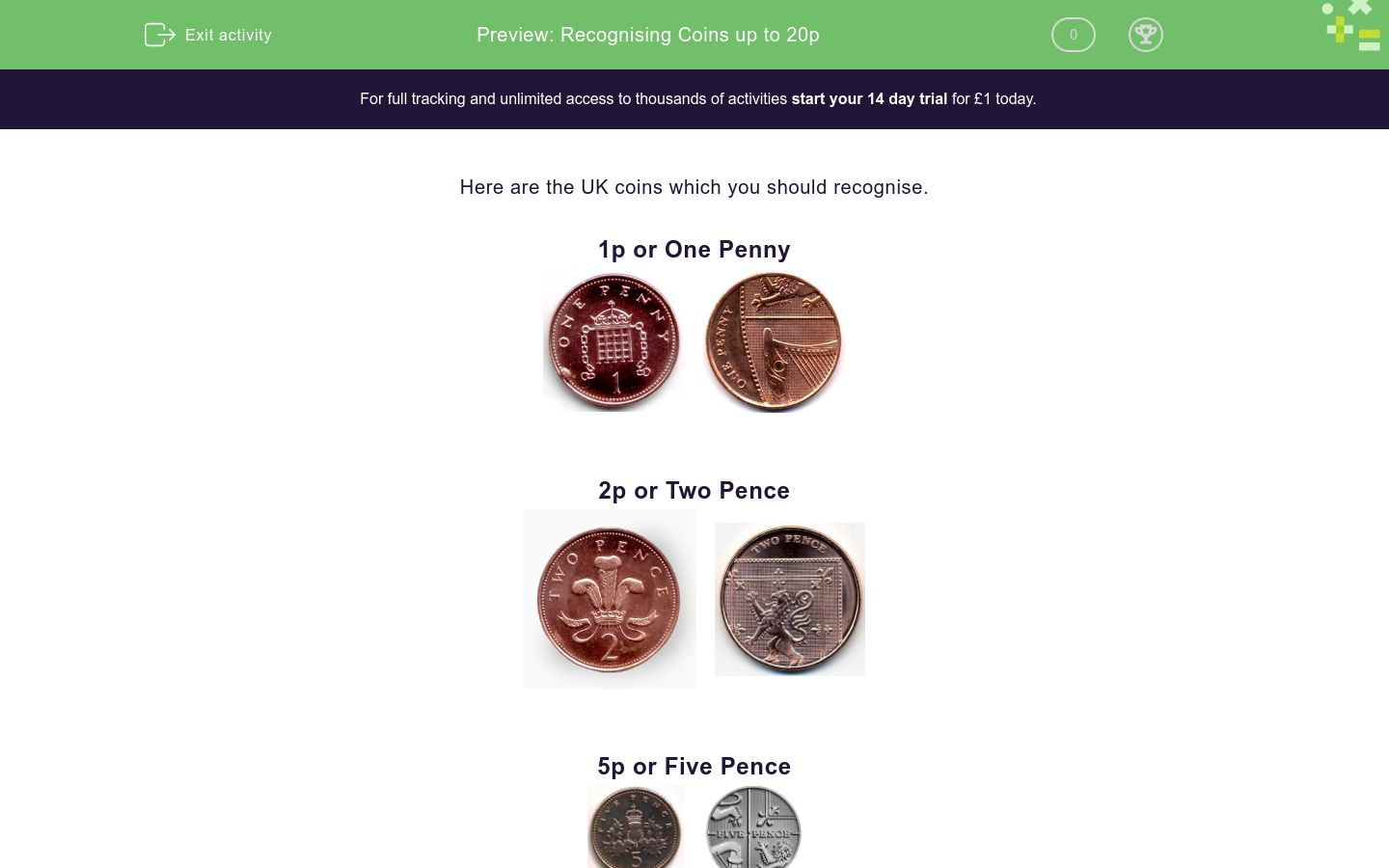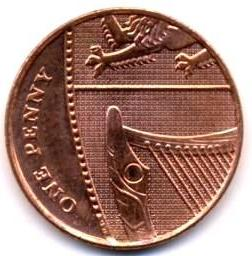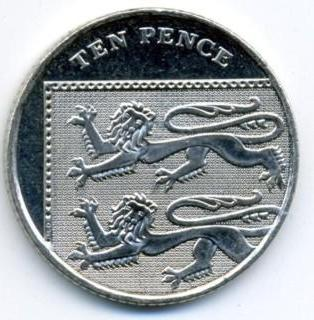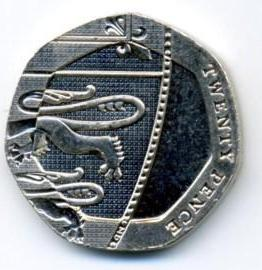# Recognising Coins up to 20p

In this worksheet, students must recognise coins up to and including 20p coins.Key stage:  KS 1

Curriculum topic:   Measurement

Curriculum subtopic:   Money and its Value

Difficulty level:### QUESTION 1 of 10

Here are the UK coins which you should recognise.

1p or One Penny2p or Two Pence5p or Five Pence10p or Ten Pence20p or Twenty PenceHow much is this coin worth?1p

2p

5p

10p

20p

How much is this coin worth?1p

2p

5p

10p

20p

How much is this coin worth?1p

2p

5p

10p

20p

How much is this coin worth?1p

2p

5p

10p

20p

How much is this coin worth?1p

2p

5p

10p

20p

How much is this coin worth?1p

2p

5p

10p

20p

How much is this coin worth?1p

2p

5p

10p

20p

How much is this coin worth?1p

2p

5p

10p

20p

How much is this coin worth?1p

2p

5p

10p

20p

How much is this coin worth?1p

2p

5p

10p

20p

• Question 1

How much is this coin worth?2p
EDDIE SAYS
This is a Two Pence coin.
• Question 2

How much is this coin worth?5p
EDDIE SAYS
This is a Five Pence coin. The clue in this picture is the number 5, did you spot that?
• Question 3

How much is this coin worth?1p
EDDIE SAYS
This is a One Penny coin. Did you look for the number to give you a clue? Our different coins are also different colours, if the coin is bronze it must be a 1p or a 2p.
• Question 4

How much is this coin worth?20p
EDDIE SAYS
Are you getting the hang of this now? There are several key things to look out for, the colour, the shape and the number! This is a Twenty Pence coin.
• Question 5

How much is this coin worth?10p
EDDIE SAYS
This is a Ten Pence coin.
• Question 6

How much is this coin worth?5p
EDDIE SAYS
This is a Five Pence coin.
• Question 7

How much is this coin worth?1p
EDDIE SAYS
This is a One Penny coin. This coin does not have a number written on it, but if you look carefully you will see it says 'one penny' on it.
• Question 8

How much is this coin worth?2p
EDDIE SAYS
Did you read the coin this time? This is a Two Pence coin.
• Question 9

How much is this coin worth?10p
EDDIE SAYS
What does the writing on the coin say? This is a Ten Pence coin.
• Question 10

How much is this coin worth?20p
EDDIE SAYS
The 20p coin is quite an unusual shape, so you should look at this first, and if in doubt have a look at the wording! What shape is it? It's a heptagon! This is a Twenty Pence coin.
---- OR ----

Sign up for a £1 trial so you can track and measure your child's progress on this activity.

### What is EdPlace?

We're your National Curriculum aligned online education content provider helping each child succeed in English, maths and science from year 1 to GCSE. With an EdPlace account you’ll be able to track and measure progress, helping each child achieve their best. We build confidence and attainment by personalising each child’s learning at a level that suits them.

Get started